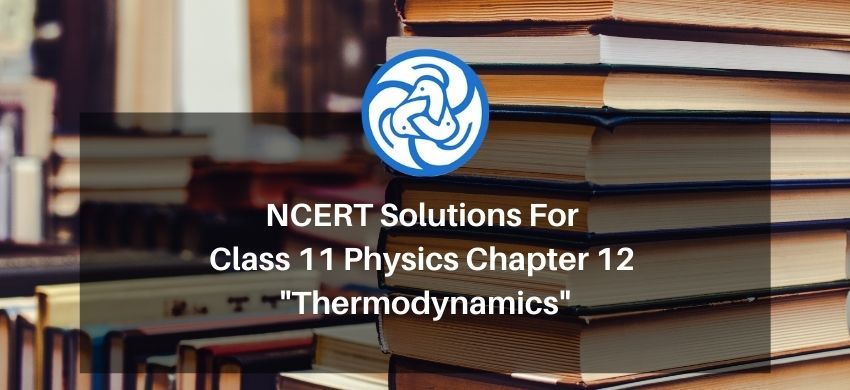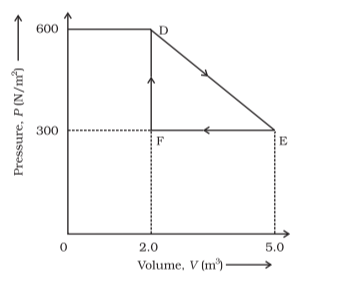# NCERT Solutions for Class 11 Physics chapter 12 - Thermodynamics - Free PDF downloadHey, are you a class 11 student and looking for ways to download NCERT Solutions for Class 11 Physics chapter 12 "Thermodynamics"? If yes. Then read this post till the end.

In this article, we have listed NCERT solution for class 11 Physics Chapter 12 in PDF that are prepared by Kota’s top IITian’s Faculties by keeping Simplicity in mind.

If you want to learn and understand class 11 Physics chapter 12 "Thermodynamics" in an easy way then you can use these solutions PDF.

NCERT Solutions helps students to Practice important concepts of subjects easily. Class 11 Physics solutions provide detailed explanations of all the NCERT questions that students can use to clear their doubts instantly.

If you want to score high in your class 11 Physics Exam then it is very important for you to have a good knowledge of all the important topics, so to learn and practice those topics you can use eSaral NCERT Solutions.

So, without wasting more time Let’s start.

### Download The PDF of NCERT Solutions for Class 11 Physics Chapter 12 "Thermodynamics"

Question 1. A geyser heats water flowing at the rate of $3.0$ litres per minute from $27^{\circ} \mathrm{C}$ to $77^{\circ} \mathrm{C}$. If the geyser operates on a gas burner, what is the rate of consumption of the fuel if its heat of combustion is $4.0 \times 10^{4} \mathrm{~J} / \mathrm{g}$ ?

Solution. Water is flowing at a rate of $3.0$ litre/min.

When the geyser heats the water, the temperature is raised from $27^{\circ} \mathrm{C}$ to $77^{\circ} \mathrm{C}$.
Initial temperature, $T_{1}=27^{\circ} \mathrm{C}$
Final temperature, $T_{2}=77{ }^{\circ} \mathrm{C}$
$\therefore$ Rise in temperature, $\Delta T=T_{2}-T_{1}=77-27=50^{\circ} \mathrm{C}$
The heat of combustion $=4 \times 10^{4} \mathrm{~J} \mathrm{~g}$
Specific heat of water, $c=4.2 \mathrm{~g}^{-1} \mathrm{c}^{-1}$
Mass of flowing water, $\mathrm{m}=\frac{3.0 \text { litre }}{\min }=3000 \mathrm{~g} / \mathrm{min}$
Total heat used, $\Delta Q=m c \Delta T=300 \times 4.2 \times 50=6.3 \times 10^{5} \frac{\mathrm{J}}{\mathrm{min}}$
$\therefore$ Rate of consumption $=\frac{6.3 \times 10^{5}}{4 \times 10^{4}}=15.75 \mathrm{~g} / \mathrm{min}$

Question 2. What amount of heat must be supplied to $2.0 \times 10^{-2} \mathrm{~kg}$ of nitrogen (at room temperature) to raise its temperature by $45^{\circ} \mathrm{C}$ at constant pressure? (Molecular mass of $\mathrm{N}_{2}=28 ; \mathrm{R}=8.3 \mathrm{~J} \mathrm{~mol}^{-1} \mathrm{~K}^{-1}$.)

Solution:
Mass of nitrogen, $m=2.0 \times 10^{-2} \mathrm{~kg}=20 \mathrm{~g}$
Rise in temperature, $\Delta T=45^{\circ} \mathrm{C}$
Molecular mass of $N_{2}, M=28$
Universal gas constant, $R=8.3 \mathrm{~J} \mathrm{~mol}^{-1} \mathrm{~K}^{-1}$
$n=\frac{m}{M}$
Number of moles, $n=\frac{m}{M}$
$=\frac{2.0 \times 10^{-2} \times 10^{3}}{28}=0.714$
Molar specific heat for nitrogen at constant pressure, $C_{p}=\frac{7}{2} R$
$=\frac{7}{2} \times 8.3$
The total amount of heat to be supplied is:
$\Delta Q=n C_{p} \Delta T$
$=0.714 \times 29.05 \times 45$
$=933.38 \mathrm{~J}$
Thus, the amount of heat to be supplied is $933.38 \mathrm{~J}$

Question 3. Explain why
(a) Two bodies at different temperatures $T_{1}$ and $T_{2}$ if brought in thermal contact do not necessarily settle to the mean temperature $\frac{\left(T_{1}+T_{2}\right)}{2}$.
(b) The coolant in a chemical or a nuclear plant (i.e., the liquid used to prevent the different parts of a plant from getting too hot) should have high specific heat.
(c) Air pressure in a car tyre increases during driving.
(d) The climate of a harbour town is more temperate than that of a town in a desert at the same latitude.

Solution: (a) Till equilibrium is achieved, heat flows from the body at the higher temperature to the body at the lower temperature when two bodies at different temperatures $\mathrm{T}_{1}$ and $\mathrm{T}_{2}$ are brought in thermal contact, i.e., the temperatures of both the bodies become the same. The equilibrium temperature is the same as the mean temperature $\frac{\mathrm{T}_{1}+\mathrm{T}_{2}}{2}$ only when the thermal capacities of both bodies are equal.
(b) The specific heat should be high for the coolant used in a chemical or nuclear plant. This is because if the specific heat of the coolant is higher, its heat-absorbing capacity is also higher and vice versa. Hence, the best coolant to be used in a nuclear or chemical plant is a liquid having a high specific heat is. This would prevent different parts of the plant from getting too hot.
(c) When a car is in motion, because of the motion of the air molecules, the air temperature inside the car increases. According to Charles' law, the temperature is directly proportional to pressure. Hence, the air pressure in the tire will also increase if the temperature inside a tyre increases.
(d) A harbour town has a more temperate climate (i.e., without the extremes of heat or cold) than a town located in a desert at the same latitude. This is because the relative humidity in a harbour town is more than it is in a desert town.

Question 4. A cylinder with a movable piston contains 3 moles of hydrogen at standard temperature and pressure. The walls of the cylinder are made of a heat insulator, and the piston is insulated by having a pile of sand on it. By what factor does the pressure of the increase if the gas is compressed to half its original volume?

Solution. The cylinder is insulated completely from its surroundings. Thus, no heat is exchanged between the system (cylinder) and its surroundings. Thus, the process is adiabatic.
Initial pressure inside the cylinder $=P_{1}$
Final pressure inside the cylinder $=P_{2}$
The initial volume inside the cylinder $=V_{1}$
The final volume inside the cylinder $=V_{2}$
The ratio of specific heats, $\mathrm{Y}=1.4$
For an adiabatic process, we have:
$P_{1} V_{1}^{\gamma}=P_{2} V_{2}^{\gamma}$
The final volume is compressed to an amount equal to half of its initial volume.
$V_{2}=\frac{V_{1}}{2}$
$P_{1}\left(V_{1}\right)^{\gamma}=P_{2}\left(\frac{V_{1}}{2}\right)^{\gamma}$
$\frac{P_{2}}{P_{1}}=\frac{\left(V_{1}\right)^{\gamma}}{\left(\frac{V_{1}}{2}\right)^{T}}=(2)^{\gamma}=(2)^{1,4}=2.639$
Thus, the pressure increases by a factor of $2.639$.

Question 5. In changing the state of a gas adiabatically from an equilibrium state $A$ to another equilibrium state $B$, an amount of work equal to $22.3 \mathrm{~J}$ is done on the system. If the gas is taken from state $A$ to $B$ via a process in which the net heat absorbed by the system is $9.35 \mathrm{cal}$, how much is the net work done by the system in the latter case ? (Take $1 \mathrm{cal}=4.19 \mathrm{~J}$ )

Solution. iIven
While the gas changes from state $A$ to state $B$, the work done $(\mathrm{W})$ on the system is $22.3 \mathrm{~J}$. This is an adiabatic process. Hence, the change in heat is zero.
$\therefore \Delta Q=0$
$\Delta W=-22.33$ (As the work is done on the system)
Using the first law of thermodynamics, we have:
$\Delta Q=\Delta U+\Delta W$
Where,
$\Delta \mathrm{U}=\mathrm{Change}$ in the internal energy of the gas
$\therefore \Delta U=\Delta Q-\Delta W=-(-22.3)$
$\Delta U=+22.3 \mathrm{~J}$
The net heat absorbed by the system when the gas goes from state $\mathrm{A}$ to state $\mathrm{B}$ via a process is:
$\Delta Q=9.35 \mathrm{cal}=9.35 \times 4.19=39.1765 \mathrm{~J}$
The heat absorbed, $\Delta Q=\Delta U+\Delta Q$
$\Delta W=\Delta Q-\Delta U$
$=39.1765-22.3$
$=16.8765 \mathrm{~J}$

Question 6. Two cylinders $A$ and $B$ of equal capacity are connected to each other via a stopcock. A contains gas at standard temperature and pressure. $B$ is completely evacuated. The entire system is thermally insulated. The stopcock is suddenly opened. Answer the following:
(a) What is the final pressure of the gas in $A$ and $B ?$
(b) What is the change in internal energy of the gas?
(c) What is the change in the temperature of the gas?
(d) Do the intermediate states of the system (before settling to the final equilibrium state) lie on its $P-V-T$ surface?

Solution:(a) $0.5 \mathrm{~atm}$
As soon as the stopcock is opened between cylinders $A$ and $B$, the volume available to the gas is doubled. As the volume and pressure are inversely proportional, the pressure will decrease to half of the original value. As the initial pressure of the gas is $1 \mathrm{~atm}$, the pressure in each cylinder will be $0.5$ atm.
(b) zero
Only when work is done by or on the gas, the internal energy of the gas can change. The internal energy of the gas won't change since in this case no work is done by or on the gas.
(c) Zero
The temperature of the gas won't change at all as, during the expansion of the gas, no work is being done by the gas.
(d) No
The free expansion is rapid, and it cannot be controlled. The intermediate states of the process do not satisfy the gas equation, and they do not lie on the P-V-T surface of the system since they are in non-equilibrium states.

Question 7. A steam engine delivers $5.4 \times 10^{8} \mathrm{~J}$ of work per minute and services $3.6 \times 10^{9} \mathrm{~J}$ of heat per minute from its boiler. What is the efficiency of the engine? How much heat is wasted per minute?

Solution. Work done per minute by the steam engine
$\mathrm{W}=5.4 \times 10^{8} \mathrm{~J}$
The heat supplied from the boiler, $\mathrm{H}=3.6 \times 10^{9} \mathrm{~J}$
Output energy
The efficiency of the engine $=$ Input energy
$\therefore \eta=\frac{W}{H}=\frac{5.4 \times 10^{8}}{3.6 \times 10^{9}}=0.15$
Thus, the percentage efficiency of the engine is $15 \%$.
The amount of heat wasted $=3.6 \times 10^{9}-5.4 \times 10^{8}$
$=30.6 \times 10^{8} \mathrm{~J}=3.06 \times 10^{9} \mathrm{~J}$
Thus, the amount of heat wasted per minute is $3.06 \times 10^{9} \mathrm{~J}$.

Question 8. An electric heater supplies heat to a system at a rate of $100 \mathrm{~W}$. If the system performs work at a rate of 75 joules per second. At what rate is the internal energy increasing?

Solution. The heat is supplied to the system at a rate of $100 \mathrm{~W}$.
The heat supplied, $\mathrm{Q}=100 \mathrm{~J} / \mathrm{s}$
The system performs work at a rate of $75 \mathrm{~J} / \mathrm{s}$.
Work done, $\mathrm{W}=75 \mathrm{~J} / \mathrm{s}$
According to the first law of thermodynamics, we have:
$Q=U+W$
Where,
$U=$ Internal energy
$\therefore \mathrm{U}=\mathrm{Q}-\mathrm{W}$
$=100-75$

$=25 \mathrm{~J} / \mathrm{s}$
$=25 \mathrm{~W}$
Thus, the internal energy of the given electric heater increases at a rate of $25 \mathrm{~W}$.

Question 9. A thermodynamic system is taken from an original state to an intermediate state by the linear process shown in Fig. (12.13)Its volume is then reduced to the original value from $\mathrm{E}$ to $\mathrm{F}$ by an isobaric process.
Calculate the total work done by the gas from $D$ to $E$ to $F$.

Solution. The total work done by the gas from $\mathrm{D}$ to $\mathrm{E}$ to $\mathrm{F}=$ Area of $\triangle \mathrm{DEF}$ Area of $\triangle \mathrm{DEF}=$ $\frac{1}{2} \mathrm{DE} \times \mathrm{EF}$
Where,
$\mathrm{DF}=$ Change in pressure
$=600 \mathrm{~N} / \mathrm{m}^{2}-300 \mathrm{~N} / \mathrm{m}^{2}$
$=300 \mathrm{~N} / \mathrm{m}^{2}$
The change in volume,
$\mathrm{EF}=5.0 \mathrm{~m}^{3}-2.0 \mathrm{~m}^{3}$
$=3.0 \mathrm{~m}^{3}$
Area of $\Delta \mathrm{DEF}=\frac{1}{2} \times 300 \times 3$
Thus, the total work done by the gas from $D$ to $E$ to $F$ is $450 \mathrm{~J}$.

Question 10. A refrigerator is to maintain eatables kept inside at $9{ }^{\circ} \mathrm{C}$. If the room temperature is $36^{\circ} \mathrm{C}$, calculate the coefficient of performance.

Solution:The temperature inside the refrigerator, $\mathrm{T}_{1}=9{ }^{\circ} \mathrm{C}=282 \mathrm{~K}$
Room temperature, $\mathrm{T}_{2}=36{ }^{\circ} \mathrm{C}=309 \mathrm{~K}$
$\frac{\mathrm{T}_{1}}{\mathrm{~T}_{2}-T_{1}}$
$=\frac{282}{309-282}$
$=10.44$
Thus, the coefficient of performance of the refrigerator is $10.44$.